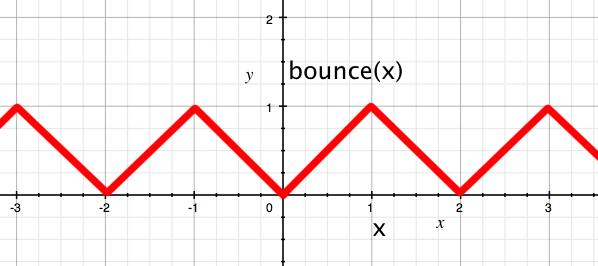number of particles

## Web gl particle box

### by Cody Smtih

This is a non-interacting particle simulator inspired by Ed Angels OpenGL example. Basically all that a particle can do is move in a straight line. but some trickery makes it look like it bounces around. Since its just a shader trick it can handle 20,000, or more, particles very easily.

### how it works

every particle starts with an initial position and velocity. Modulo arithmatic allows the particle to bounce off of walls.here is a graph of the bounce function that makes the particles appear to bounce off walls. It is applied to the x,y, and z positions

```    attribute vec3 aVertexPosition;
attribute vec4 aVertexColor;
attribute vec3 velocity;

uniform mat4 uPMatrix;
uniform mat4 viewMatrix;
uniform float timeStep;

varying vec4 vColor;

float bounce( float x)
{
float xa = abs(x);
float x2 = mod(xa,2.0);
float x3 = mod(xa,1.0);
if( x2 > 1.0){
x3 = 1.0 - x3;
}
return x3;
}

void main(void) {

vec3 b =  vec3( aVertexPosition - ( timeStep * velocity));
gl_Position = vec4(bounce(b.x), bounce(b.y), bounce(b.z), 1.0);

gl_Position = viewMatrix * gl_Position;
gl_Position = uPMatrix * gl_Position;
vColor = aVertexColor;
//vColor = vec4( velocity, 1.0);
}
```

### what i learned:

The trackball taught me a lot about quaternions. Some of which I understand. for example i,j,k are all different imaginary numbers. There is a multiplaication table that was invented for them that allows them to actually work. it also is very cool technique.

### improvements:

Add a bounding box to make it visually appealing. Add slider to change number of particles.

### limitations:

I don't see an easy way to allow inter-particle interactions. seems to be limited to a cube environment. probably difficult to implement gravity or any other forces.